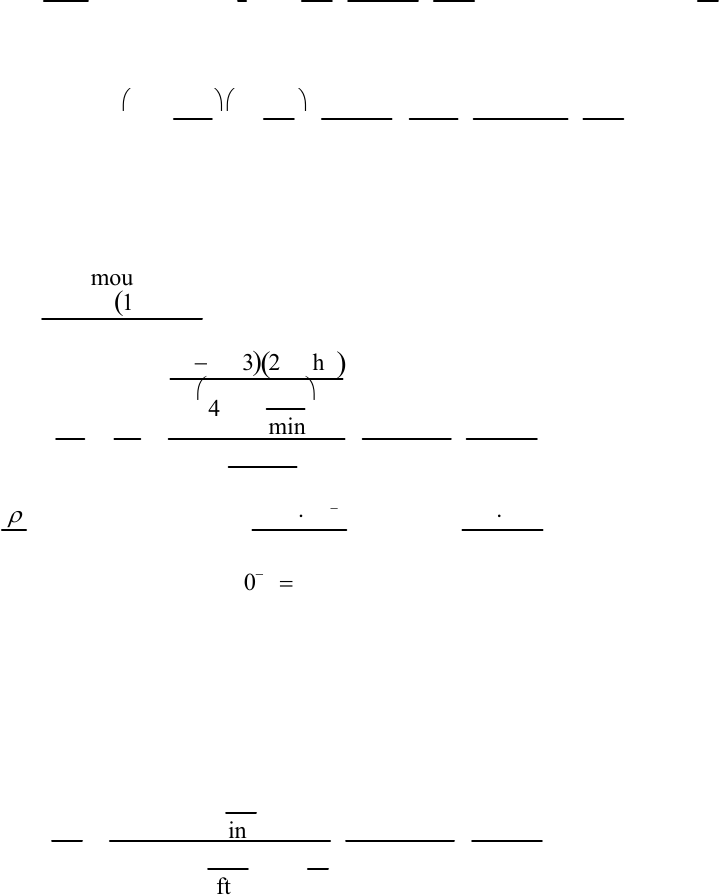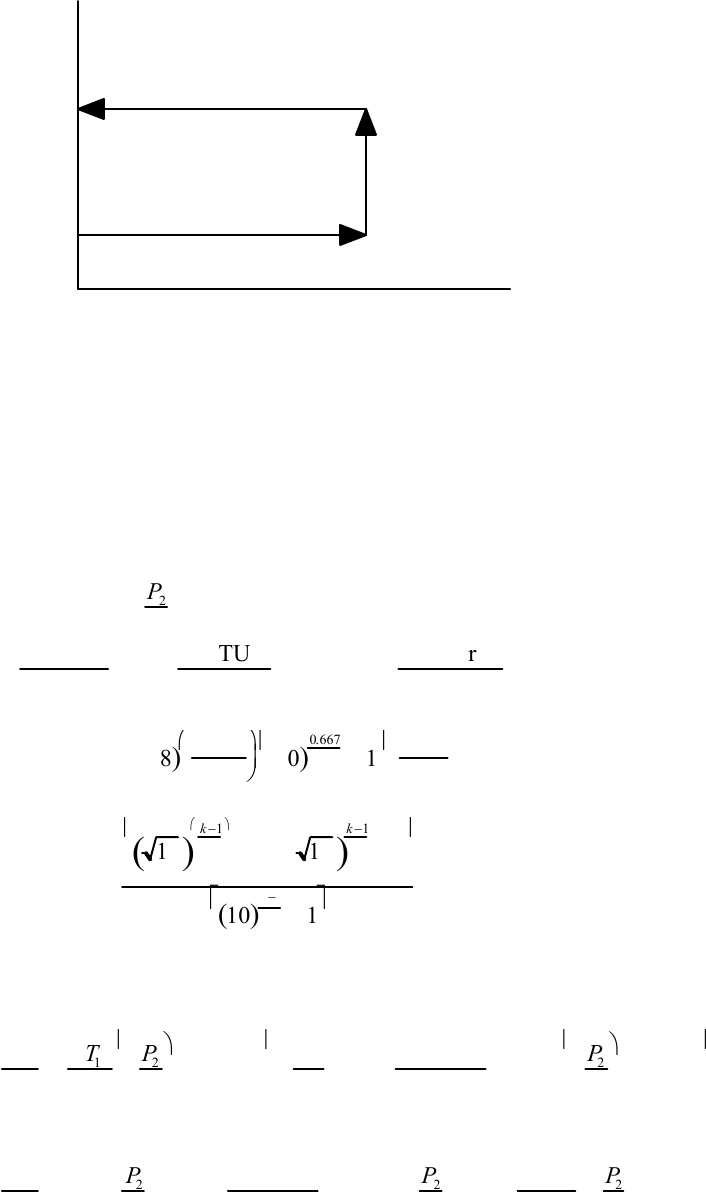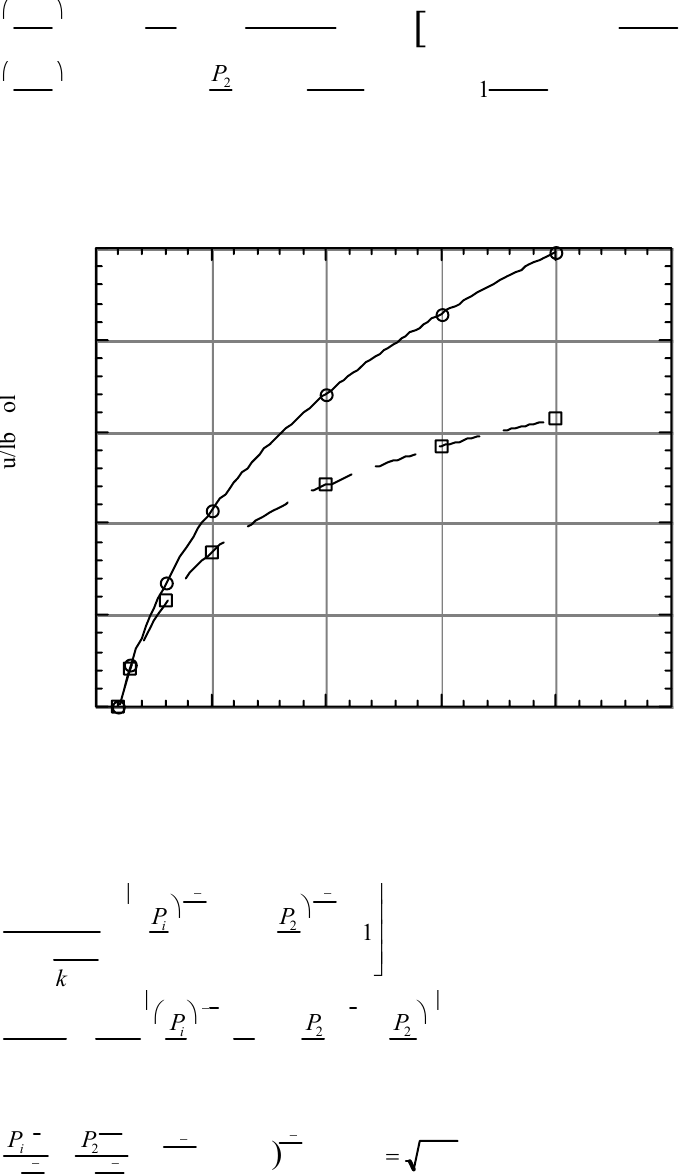Study Guides (400,000)
US (230,000)
LSU (10,000)
CHE (50)
All (10)
Final

# Ch 10Exam

Department
Chemical Engineering
Course Code
CHE 3101
Professor
All
Study Guide
Final

This preview shows pages 1-3. to view the full 12 pages of the document.Solutions, Chapter 10
______________________________________________________________________
10.1* Q=AL
t=10 in25in1
s=50 in3
sgal
231 in360 s
min =13.0 gpm = 8.2 10-4 m
s
______________________________________________________________________
10.2 Po =QΔP=500 gal
min
25 lbf
in2
231 in3
gal ft
12 in hp s
550 ft lbf min
60 s
=
7.29 hp = 5.44 kW
This answer is independent of fluid properties for a constant-density fluid.
______________________________________________________________________
10.3 (a) The amount of energy converted to friction heating is
F=2.8 hp 10.73
(
)
Ý
m u
ΔT=Δu
CV
=F
CV
=
10.73
()280 hp
(
)
416.5 lbm
min
1Btu
lbm oF
2545 Btu
hp hr hr
60 min =0.077oF = 0.042°C
(b)
ρ
ρ
0
=1
α
ΔT+
β
ΔP=10.11
10
3
°F0.077°F+0.3
10-5
psi 70 psi
=18.5 106+2.110
4
=
1.0002
In this case the friction heating is very small, so its effect is less than that of the increase
in pressure. The two effects act in opposite directions. The resulting change in density is
certainly negligible.
______________________________________________________________________
10.4 See Ex. 10.2. At room temperature the vapor pressure of mercury is 10-6 atm
0, so that
Δz=ΔP
ρ
g=
14.7 lbf
in2
13.6 62.3 lbm
f
t
332.2 ft
s2
32.2 lbm ft
lbf s2144 in2
ft2=2.50 ft = 0.76 m
______________________________________________________________________
10.5 One regularly sees Fig 10.6 or its equivalent, but rarely sees the same figure for
an incompressible fluid, because it is so simple. It is shown below. Asking students to
sketch it helps them understand Fig. 10.6.
Solutions, Fluid Mechanics for Chemical Engineers, Third Edition, Chapter 10, page 1

Only pages 1-3 are available for preview. Some parts have been intentionally blurred.P
V
in
out
______________________________________________________________________
10.6 Differential thermal expansion between the various parts of the compressor make it
impractical. The cylinder walls and head are cooled, the piston is generally not. Some
clearance room must be allowed for this.
______________________________________________________________________
10.7* (a)Po =Ý
n RT ln P2
P
1
=20 lbmol
hr 1.987 BT
U
lbmol oR528 oRln10 hp h
r
2545 BTU =18.98 hp =14.16 kW
(b)
=20()1.987()528()
1.667
0.667
10
()
0.667
1.667 1
1
2545 =31.16 hp =23.2 kW
(c) Po =above
10
()
k1
k
1+10
()
k1
k1
10
()
k1
k1
=24.11 hp = 18.0 kW
______________________________________________________________________
ΔW
n=RT
1k
k1
P
2
P
1
(k1)/k
1
=1.4
0.4 1.987 Btu
lbmol °R528°RP
2
P
1
0.4 /1.4
1
While for an isothermal compressor
ΔW
n=RT ln P
2
P
1
=1.987 Btu
lbmol °R528°RlnP2
P
1
=1043 Btu
lbmol ln P
2
P
1
For
P
2/
P
1=20
Solutions, Fluid Mechanics for Chemical Engineers, Third Edition, Chapter 10, page 2

Only pages 1-3 are available for preview. Some parts have been intentionally blurred.ΔW
n
=1.4
0.4 1.987 Btu
lbmol °R528°R20()
0.4/1.4 1
[
]=4968 Btu
lbmol
Δ
W
n
isothermal
=RT ln P
2
P
1
=1043 Btu
lbmol ln20 =3141 Btu
lbmol
Using a spreadsheet we compute the corresponding values for intermediate pressures and
plot them as shown below. As expected, the isothermal always has a lower power
0
1000
2000
3000
4000
5000
0 5 10 15 20 25
P / P
21
W/n, Btu
/lb
mo
l
Isothermal
______________________________________________________________________
10.9 ΔW
η
RT
1
k
k
1
=P
i
P
1
k1
k1+P
2
P
i
k
1
k1
detc.()
dP
i
=k+1
k
P
i
P
1
1
k1
P
1
+P
2
Pi
1
kP
2
P
i
2
We set this equal to zero, and
Pi
1
k
P
1
k1
k
=P
2
k1
k
P
i
2k1
k
; P
i
2k2
k=P
1P
2
()
k
1
k; P
i
=
P
1P
2
______________________________________________________________________
Solutions, Fluid Mechanics for Chemical Engineers, Third Edition, Chapter 10, page 3